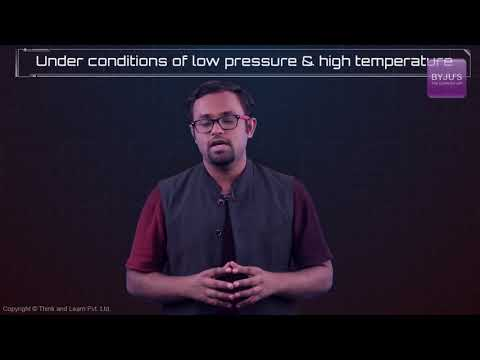# Derivation of Ideal Gas Equation

## What is Ideal Gas?

An ideal gas is a theoretical gas composed of a set of randomly-moving point particles that interact only through elastic collisions.

The ideal gas concept is useful because it obeys the ideal gas law, a simplified equation of state and is amenable to analysis under statistical mechanics.

## Recommended Videos## Ideal Gas Laws

The laws which deal with ideal gases are naturally called ideal gas laws and the laws are determined by the observational work of Boyle in the seventeenth century and Charles in the eighteenth century.

• Boyles Law – states that for a given mass of gas held at a constant temperature the gas pressure is inversely proportional to the gas volume.
• Charles Law – states that for a given fixed mass of gas held at a constant pressure the gas volume is directly proportional to the gas temperature.

## Ideal Gas Equation

The Ideal gas law is the equation of state of a hypothetical ideal gas. It is a good approximation to the behaviour of many gases under many conditions, although it has several limitations. The ideal gas equation can be written as

PV = nRT

Where,

• P is the pressure of the ideal gas.
• V is the volume of the ideal gas.
• n is the amount of ideal gas measured in terms of moles.
• R is the universal gas constant.
• T is the temperature.

According to the Ideal Gas equation-

The product of Pressure & Volume of a gas bears a constant relation with the product of Universal gas constant, number of moles of gas and  temperature.

i.e.

$$\begin{array}{l}pv = nRT\end{array}$$

## Universal Gas Constant (R)

R is a universal gas constant and it is the molar equivalent of boltzmann constant having the units of energy increased per temperature per mole. It is denoted by R.

Note: From the SI system the value of the universal gas constant is 8.314 kJ/mole.K

## Derivation of the Ideal Gas Equation

Let us consider the pressure exerted by the gas to be ‘p

The volume of the gas be – ‘v

Temperature be – “T”

“n” – be the number of moles of gas

Universal gas constant – R

According to Boyle’s Law,

At constant n & T, the volume bears an inverse relation with the pressure exerted by a gas.

i.e.

$$\begin{array}{l}v \propto \frac{1}{p}\end{array}$$
………………………………(i)

According to Charles’ Law,

When p & n are constant, the volume of a gas bears a direct relation with the Temperature.

i.e.

$$\begin{array}{l}v \propto T\end{array}$$
………………………………(ii)

When p & T are constant, then the volume of a gas bears a direct relation with the number of moles of gas.

i.e.

$$\begin{array}{l}v \propto n\end{array}$$
………………………………(iii)

Combining all the three equations, we have-

$$\begin{array}{l}v \propto \frac{nT}{p}\end{array}$$

or

$$\begin{array}{l}pv = nRT\end{array}$$

where R is the Universal gas constant, which has a value of 8.314 J/mol-K

## Frequently Asked Questions – FAQs

### What is ideal gas equation and derive it mathematically?

The ideal gas equation is formulated as: PV = nRT. In this equation, P refers to the pressure of the ideal gas, V is the volume of the ideal gas, n is the total amount of ideal gas that is measured in terms of moles, R is the universal gas constant, and T is the temperature.

### What is meant by ideal gas equation?

An equation that equates the product of the pressure and the volume of one mole of a gas to the product of its thermodynamic temperature and the gas constant. The equation is exact for an ideal gas and is a good approximation for real gases at low pressures. Also called: ideal gas equation, ideal gas law.

### What is ideal gas example?

Many gases such as nitrogen, oxygen, hydrogen, noble gases, some heavier gases like carbon dioxide and mixtures such as air, can be treated as ideal gases within reasonable tolerances over a considerable parameter range around standard temperature and pressure.

### What is an ideal gas explain?

An ideal gas is defined as one in which all collisions between atoms or molecules are perfectly eleastic and in which there are no intermolecular attractive forces. In such a gas, all the internal energy is in the form of kinetic energy and any change in internal energy is accompanied by a change in temperature.

### What are ideal gas conditions?

For a gas to be “ideal” there are four governing assumptions: The gas particles have negligible volume. The gas particles are equally sized and do not have intermolecular forces (attraction or repulsion) with other gas particles. The gas particles have perfect elastic collisions with no energy loss.

Test your Knowledge on Derivation of ideal gas equation!

1. Nice